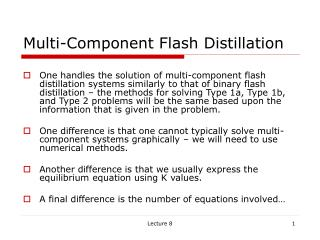# Multi-Component Flash Distillation - PowerPoint PPT PresentationDownload PresentationMulti-Component Flash Distillation

Multi-Component Flash DistillationDownload Presentation## Multi-Component Flash Distillation

- - - - - - - - - - - - - - - - - - - - - - - - - - - E N D - - - - - - - - - - - - - - - - - - - - - - - - - - -
##### Presentation Transcript

1. Multi-Component Flash Distillation • One handles the solution of multi-component flash distillation systems similarly to that of binary flash distillation – the methods for solving Type 1a, Type 1b, and Type 2 problems will be the same based upon the information that is given in the problem. • One difference is that one cannot typically solve multi-component systems graphically – we will need to use numerical methods. • Another difference is that we usually express the equilibrium equation using K values. • A final difference is the number of equations involved… Lecture 8

2. Multi-Component Flash Distillation – How many unknowns? • Suppose we have 10 components: • For a 10 component problem, C = 10, assuming that F, zi’s for C-1 components, Pd, and Td or one of the xi’s or yi’s are specified (a Type 1a Problem), we can solve the equilibrium and mass balances first and then the energy balance (a sequential solution) • However, we still need to determine 10 K’s, 10 x’s, 10 y’s, 1 L, and 1 V, or 32 variables! Lecture 8

3. Multi-Component Flash Distillation – How many equations? • 32 Equations: Lecture 8

4. Multi-Component Flash Distillation – How do we readily handle? • We could define all 32 equations, and solve the 32 simultaneous equations simultaneously. • This may or may not be possible – we are dealing with a large number of simultaneous equations, and one may experience convergence problems in a numerical solution. • How can we “help” the convergence? One method is to partially solve the set of equations for a parameter which is tightly bounded – that is its values cannot vary widely. • A convenient choice is the fraction of feed vaporized, f = V/F, which varies between 0 and 1. Lecture 8

5. Multi-Component Flash Distillation – Combining Equilibrium Relationship and Mass Balances Lecture 8

6. Multi-Component Flash Distillation – Rachford-Rice Equation Lecture 8